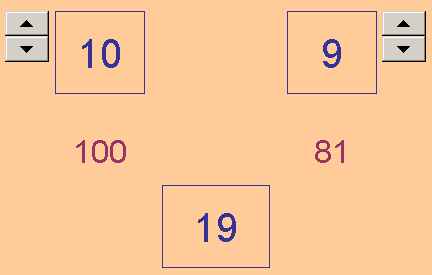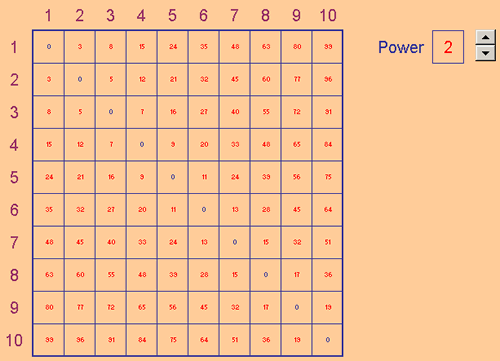# Excel Investigation: the Difference of Two (same) Powers

### Starting with Squares:

If you take two integers and look at the difference between the square of each value, there is a nice relationship between the original numbers and that difference.

The Excel file Difference of Two Squares.xls lets you explore this.( Right-click on the link, "Save Target As", and select where you want the file to be saved.)Sometimes a table of values helps, and the Excel file As a Table.xls gives you that.

Look at the numbers and see if you spot anything that might be helpful.

For example under the 6 you'll see 35, 32, 27 and 20 (notice anything?).

If you know about the Difference of Two Squares already then move straight on to Beyond Squares.

### How to make the Table:

In the file "As a Table", the formula in D3 is: = ABS ( $C3 ^ 2 - D$2 ^ 2 ).

This calculates the (positive) difference between C3 squared and D2 squared.

The dollar sign on the C of C3 means that if this formula is copied elsewhere the C part of the cell reference will not be adjusted for the new position. The formula will always pick up its first value from column C. In the same way the dollar sign on the 2 of D2 means that when this formula is copied to new positions the cell reference will keep with row 2.

Once this formula has been entered in D3 , right-click on D3 and choose Copy, select the whole table including D3, right-click and Paste.

### Beyond Squares:

You may have discovered that when the original numbers differ by 2 the difference of their squares always contains a factor of 2, but when the original numbers differ by 3, the difference of their squares contains a factor of 3, and so on. Of course the important thing to do when you reach that point is to understand why this is true.

After that, a natural extension to this investigation would be to try other powers. When the original numbers differ by 2 does the difference of the cubes have 2 as a factor? Is the difference of fourth powers also a multiple of 2 ? And so on.

In general : is an - bn always a multiple of a - b for all positive integer values of n?

Here's an Excel sheet to give you some useful results as you think about this (with a spinner to set the power from 2 to 6) Table of Powers.xls :The cell D3 contains a very similar formula to that used in the "As aTable" file earlier but the power is the value in cell P3 instead of plain 2.

We don't really want to check each number in the table to see whether it's a multiple of the difference between its two source values.

Conditional Formatting is perfect for this job. Excel does the check for us and uses a colour change to indicate the outcome of that test.

Conditional Formatting using a formula : Select D3 and choose Conditional Formatting from the Format drop-down menu.

You will see the following formula = MOD ( D3, ABS ( D$2-$C3 ) ) = 0.

This tests to see if the cell, D3, when divided by the difference between D2 and C3, gives a remainder of zero.

In other words, it tests whether D3 is a multiple of ABS ( D2 - C3 ). The font colour changes from blue to red when this condition is satisfied - it seems that it always is.

So it looks like an - bn , where n is a positive integer, is always a multiple of a - b , but can we see why?

This is not easy to do, but is a very interesting result.

Perhaps try explaining this for n = 3 , then n = 4 , and so on. If you do manage that, you are just one step away from seeing what the other factor must be. For the difference of two squares the factors were (a - b) and (a + b) For the difference of two cubes the factors are (a - b) and ( ? ? ? ) For the difference of two fourth powers the factors are (a - b) and ( ? ? ? ? ). What is the general result?

If you get this far you really are doing some serious investigating.